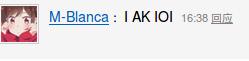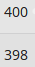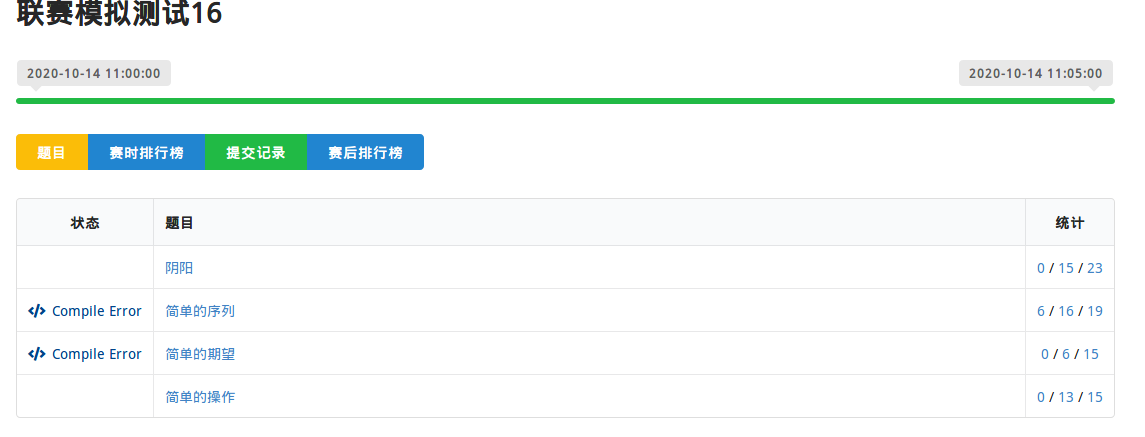# Diary（2020十月 CSP赛前集训）

### $Sublime$

wget files-cdn.cnblogs.com/files/rui-4825/sub-set.sh
sh sub-set.sh


## 2020.11.01-11.06

### 2020.11.05(1)

$\Huge 1$

//https://item.jd.com/100000914807.html#crumb-wrap

### 2020.11.04(2)

$\Huge 2$

### 2020.11.03(3)

$\Huge 3$

### 2020.11.02(4)

$\Huge 4$

，，，，

1、程序员喝啤酒
大家喝的是啤酒，这时你入座了。
你给自己倒了杯可乐，这叫低配置。
你给自已倒了杯啤酒，这叫标准配置。
你给自己倒了杯茶水，这茶的颜色还跟啤酒一样，这叫木马
你给自己倒了杯可乐，还滴了几滴醋，不仅颜色跟啤酒一样，而且不冒热气还有泡泡，这叫超级木马。
你的同事给你倒了杯白酒，这叫推荐配置。
菜过三巡，你就不跟他们客气了。
你向对面的人敬酒，这叫 p2p。
你向对面的人敬酒，他回敬你，你又再敬他……，这叫 tcp。
你向一桌人挨个敬酒，这叫令牌环。
你说只要是兄弟就干了这杯，这叫广播。
有一个人过来向这桌敬酒，你说不行你先过了我这关，这叫防火墙。
你的小弟们过来敬你酒，这叫一对多。
你是 boss，所有人过来敬你酒，这叫服务器。
酒是一样的，可是喝酒的人是不同的。
你越喝脸越红，这叫频繁分配释放资源。
你越喝脸越白，这叫资源不释放。
你已经醉了，却说我还能喝，叫做资源额度不足。
你明明能喝，却说我已经醉了，叫做资源保留。
喝酒喝到最后的结果都一样，你突然跑向厕所，这叫捕获异常。
你在厕所吐了，反而觉得状态不错，这叫清空内存。
你在台面上吐了，觉得很惭愧，这叫程序异常。
你在 boss 面前吐了，觉得很害怕，这叫系统崩溃。
你吐到了 boss 身上，只能索性晕倒了，这叫硬件休克。

2、系统程序员的特点
1、头皮经常发麻，在看见一个蓝色屏幕的时候比较明显，在屏幕上什幺都看不见的时候尤其明显；
2、乘电梯的时候总担心死机，并且在墙上找 reset 键
3、指甲特别长，因为按 F7 到 F12 比较省力；
4、只要手里有东西，就不停地按，以为是 Alt-F、S；
5、机箱从来不上盖子，以便判断硬盘是否在转；
6、经常莫名其妙地跟踪别人，手里不停按 F10；
7、所有的接口都插上了硬盘，因此觉得 26 个字母不够；
8、一有空就念叨“下辈子不做程序员了”；
9、总是觉得 9 号以后是a号；
10、不怕病毒，但是很害怕自己的程序；

3、如果编程语言是女人
有的女人就是 Windows 虽然很优秀，但是安全隐患太大。
有的女人就是 MFC 她条件很好，然而不是谁都能玩的起。
有的女人就是 C# 长的很漂亮，但是家务活不行。
有的女人就是C++，她会默默的为你做很多的事情。
有的女人就是汇编虽然很麻烦，但是有的时候还得求它。
有的女人就是 SQL，她会为你的发展带来莫大的帮助。

4、小红师弟和 IT 师兄的故事。
和某 wa 聊天，说阳光开朗长相美型的男生是 Mac 系统。
平易近人热情活泼的男生是 Win7。
一般的男生是 WinXP 什么的。
深度宅男就是 Dos 系统。
那么，IT 师兄应该算是 Linux 吧。
次日，小红师弟的校内签名就换成了以下内容：
“其实我喜欢像 Linux 一样的男人，能用最低调朴素的终端界面来理解我那疯狂多变得近乎偏执的命令行参数般的古怪念头；也能用 Compiz 来满足我对夸张和华丽的所有妄想；即使多用户多任务也能隔离得让我丝毫察觉不到；但是一旦让我获取了 root，一切会向我坦陈。”

5、有次某妹子和 IT 师兄约吃饭。
到了约定的地点，居然没找到人。
IT 师兄：“往南走。”
妹子：“哪边？！”
IT 师兄：“南边南边！”
妹子：“……我不知道哪里是南边。”
IT 师兄：“……你连哪里是南都不知道，还是回去好了。”
妹子：“……”妹子抑郁了。
然后，IT 师兄为表歉意，送了妹子一个……
指……南……针……

### 2020.11.01(5)

$\Huge 5$

luogu随机主题

<iframe id="asd" src="https://www.luogu.com.cn/theme/design/10000" style="width:100%;height:100%;">
</iframe>
<script>
a=document.getElementById("asd")
setInterval(()=>{
i = parseInt(Math.random() * 30000) + 100;
console.log(i)
freopen("{1:tmp}.out", "w", stdout); ]]></content> <tabTrigger>freopen</tabTrigger> </snippet>  ### 2020.10.23(14) 愉快的一天从记录开始### 2020.10.22(15) 前几天还有爆零，，， 这个阶段后两天状态还不错 （似乎自从开了个沙雕错误集锦然后就很少挂分了） 至少会的分数能拿稳了 之前心态多多少少也有点问题 缺乏自信啥的 而且每次考试对心情影响比较大 一次两次还好 成了恶性循环之后心态就有点崩 后边调整调整好多了 今天除了DSU没学熟好像题改的差不多 下午也刷了点搜索 推了几个动规 感觉还不错 下一个阶段感觉可以往上冲一冲？ 咱也不知道哪来的自信 最近似乎感觉集训挺累的 现在每天晚上一碰到床就感觉整个人沉重的不行 然后一闭上眼就昏昏沉沉的要往下坠 迷迷糊糊就睡着了 脑子无限循环的都是各种subtask、部分分、暴力 这个算法，那个技巧巴拉巴拉的 睡得还特别死 甚至昨天室友说晚上风特别大吹的窗户噼里啪啦的 我竟然一点没听见 早晨起来说做梦也记不得，说没做梦又好像脑子就没停过 自从集训之后再也没有提前醒过了哈哈哈 睡眠质量大大提升 下个阶段的整体计划还是要刷暴搜和dp 主席树啥的数据结构还是有时间或者做烦了调剂一下心情 有时候打打大码力还是蛮解压的 考场上用短时间先把所有的暴力码掉 然后再想正解挂对拍啥的 还是这样效率比较高 也比较保险最起码能少挂分甚至不挂分 不挂分+好心态=进步 还有半个月，冲冲冲  以后每天中午的歌词我尽量在放歌之前就放进去$QwQ$ 你们搁这儿吵吵啥呢缺德玩意哦吼吼原来是瑶瑶送的 历经千辛万苦终于到我手里了 大概是转了四手 怪不容易的 $/xk$ 就剩下半个月辣咱也不知道是谁给的 瑟瑟发抖 百思不得其解 这是转了多少人的手才送到 也怪不容易的 迷惑 中午问问去 今天的计划 似乎56和6都不太可更改 $DSU$ 上午学习DSU 下午改题 刷DFS&dp 联考5（不可更改） 联考6 模拟测试19&20 写总结&Diary  ### 2020.10.21(16) 震惊！我们的唉木不拉屎真的是太强辣可！三联？ 转载了一篇文章 上午考试状态感觉不错 还是尽量做到了会的分数拿满 $odk$ 那个小瑞快要回来辣！ 更新一波图集训还是蛮累的 脑子没有别的东西只有这些了 各种$Subtask$$Spj$，暴力，部分，萦绕在脑子里面，睡了一觉感觉好累哇 没有梦境就只有无限循环的几个名词 坚持，加油 ### 2020.10.20(17) 震惊！竟然有人对用户名作出这样的事情！ 题库出现了奇怪的东东说明虎哥在水，没有看题库 $xswl$ 希望人没事 晚上没有挂分唔哈哈 中午放歌了！！！ 爷青回！！ 感动到哭惹 没想到小音箱还挺给力的 虎哥圈粉～ 昨天晚上做了一晚上暴搜 今天$T1$就拿到了$20pts$暴力 分不多但挺满足 这个阶段的目标就是不挂分 虽然考的还是一如既往的糟糕 但是连着两天没有挂分就很开心 还在后面挂着，不挂分就算有进步 剩下的再慢慢来 稳一点，踏实点，别眼高手低 就按照自己的节奏 没问题的 身为一天能听七八个小时歌的用户 表示在学校将近三周听不到歌真的是太难受了 想听歌 ### 2020.10.19(18) 放好了 刚刚出去上厕所回来一个学生会喊老师好 梅 开 二 度 下午改考试题实在是改不出来 晚上找了几个$DFS$练了练 但是好像感觉还不是很强 这玩意比他妈莫队还难 眼睛有点难受 肩膀还是一如既往的疼 中午没睡好有点虚 晚安～ 今天好像没有写点东西 上午$T2$没有挂分我是非常的开心 但是$T4$不知道为什么玄学写法还水过了一个点 $T3$还是$Dfs$打挂，，， 下午$lzz$大佬给了个搜索的题单在刷 还有不知道为啥博客的主题崩掉了 然后就换回来原先的了 感觉还不错 挺简约的 忽然想攒闪存里面一些有趣的话，暂时还没想好往哪存$QwQ$ 晚点儿再说 ### 2020.10.18(19) 啊下午$luogu$水了一波月赛 下午卓卓说在学校的路上 人家都放假回来要开学了 $HZ$的假期还没到来 我可真的太开心了 电脑前面敲的肩膀疼的要命 眼睛干涩 感觉人生得到了升华 实在是太舒服辣（淦 上午联考 理解错题目然后爆肝一道题于是爆零### 2020.10.17(20) 阶段总结 啊这 又来氵总结了 虽然成绩一如既往的不可描述 但是感觉整体状态比上个阶段提升不少吧 尤其是下午把二维莫队调过了开心的不行 一个很大的问题就还是会的分数抓不住 每次都是在一点点不起眼的地方犯了一丢丢错误 于是满盘皆输 细节 还是要注重细节 再一个就是代码实现的能力亟需提升 暴搜、dp暴力短时间内还是调不出来 而且总是想骗点分数 所以说有时候在非正解上加了剪枝 企图降低代码时间开销 然后大多数就是把分数剪没了=。= 曾经剪掉15-100分不等 就还是对自己写的代码信心不足 或者没有很强的把握说这就是多少多少的部分分甚至是正解 第三就是心态这方面 整体来说感觉自己心态还算是不错的吧 但是挂分挂的多了难免心态有些爆炸 挺恶心的 磨的人都没脾气了 但还是不甘心 就不说一点都不失误 仔细算算每天少失误一点 一场考试100分总该有的吧 但凡平均下来一场100 这4天就800呢 也能处在个中间甚至偏上的水平 或者说哪天再努力一点点还能冲击第一机房？ 万一实现了呢 对吧 然后还有什么呢 哦对 什么时候把午歌落实一下QwQ 真的要清唱的话也组织一下吧 上午化学奥赛教练员也说了 今年形势确实严峻 两位教练属实不易 抽烟有害健康，老姚少抽/xyx 虎哥每天休息好，注意眼睛（每天我们都盯的眼疼） RP++ HZOI加油  我我我现在巨开心 好久没有自己调过一道题了 唔哈哈哈哈哈 二维莫队 也不过如此嘛对吧 Code #include <iostream> #include <cstring> #include <cstdio> #include <algorithm> #include <cmath> #define int long long using namespace std; inline int read(){ int x = 0, w = 1; char ch = getchar(); for(; ch > '9' || ch < '0'; ch = getchar()) if(ch == '-') w = -1; for(; ch >= '0' && ch <= '9'; ch = getchar()) x = x * 10 + ch - '0'; return x * w; } inline void write(register int x){ if(x < 0) x = ~x + 1, putchar('-'); if(x > 9) write(x / 10); putchar(x % 10 + '0'); } int s1, s2; const int ss = 205; struct node{ int lx, ly, rx, ry, id; inline bool operator < (const node &a) const { register int lx1 = (lx - 1) / s1 + 1, lx2 = (a.lx - 1) / s1 + 1; register int rx1 = (rx - 1) / s1 + 1, rx2 = (a.rx - 1) / s1 + 1; register int ly1 = (ly - 1) / s2 + 1, ly2 = (a.ly - 1) / s2 + 1; return lx1 == lx2 ? rx1 == rx2 ? ly1 == ly2 ? (ly1 & 1) ? ry < a.ry : ry > a.ry : (rx1 & 1) ? ly1 < ly2 : ly1 > ly2 : (lx1 & 1) ? rx1 < rx2 : rx1 > rx2 : lx1 < lx2; } }q; int a[ss][ss], b, cnt, s, sum, ans; inline int binary_search(register int x){ register int l = 1, r = cnt; while(l < r){ register int mid = l + r >> 1; if(b[mid] >= x) r = mid; else l = mid + 1; } return l; } inline void add1(register int u, register int l, register int r){ for(register int i = l; i <= r; i++){ sum += (s[a[u][i]] * 2 + 1); s[a[u][i]]++; } } inline void add2(register int u, register int l, register int r){ for(register int i = l; i <= r; i++){ sum += (s[a[i][u]] * 2 + 1); s[a[i][u]]++; } } inline void del1(register int u, register int l, register int r){ for(register int i = l; i <= r; i++){ sum += (1 - s[a[u][i]] * 2); s[a[u][i]]--; } } inline void del2(register int u, register int l, register int r){ for(register int i = l; i <= r; i++){ sum += (1 - s[a[i][u]] * 2); s[a[i][u]]--; } } signed main(){ freopen("vegetable.in", "r", stdin); freopen("vegetable.out", "w", stdout); register int r = read(), c = read(), Q = read(); s1 = sqrt(r), s2 = sqrt(c); for(register int i = 1; i <= r; i++) for(register int j = 1; j <= c; j++) a[i][j] = read(), b[++cnt] = a[i][j]; sort(b + 1, b + 1 + cnt); cnt = unique(b + 1, b + 1 + cnt) - b - 1; for(register int i = 1; i <= r; i++) for(register int j = 1; j <= c; j++) a[i][j] = binary_search(a[i][j]); /* for(register int i = 1; i <= r; i++, puts("")) for(register int j = 1; j <= c; j++) cout << a[i][j] << " "; */ for(register int i = 1; i <= Q; i++) q[i] = (node){read(), read(), read(), read(), i}; sort(q + 1, q + 1 + Q); register int lx = 1, ly = 1, rx = 1, ry = 1; s[a] = 1, sum = 1; for(register int i = 1; i <= Q; i++){ while(lx > q[i].lx) add1(--lx, ly, ry); while(rx < q[i].rx) add1(++rx, ly, ry); while(ry < q[i].ry) add2(++ry, lx, rx); while(ly > q[i].ly) add2(--ly, lx, rx); while(lx < q[i].lx) del1(lx++, ly, ry); while(rx > q[i].rx) del1(rx--, ly, ry); while(ry > q[i].ry) del2(ry--, lx, rx); while(ly < q[i].ly) del2(ly++, lx, rx); ans[q[i].id] = sum; } for(register int i = 1; i <= Q; i++) cout << ans[i] << '\n'; return 0; }  震惊！ 金色传说 博客园首个星星本来挺难受的 忽然感觉整个人都开心起来了 冲冲冲！ ### 2020.10.16(21) 每次都是每个题一点点小错误把分数全丢了 刚刚，下标范围打错了白给$20pts$ 是时候开个错误集锦了 $aaaaaaaaaaaaaaaaaaaaaaaaaaaaaaaaa$ $WDNMD$ 没有离散化丢了$58pts$！！！！！！！！！！ 我人都傻了于是就，，，就差两分了 给人家反杀的机会 晚上但凡挂一个点 就凉凉 吐了 昨天早晨老姚随口说了一句 “真的瘦了啊” 然后果真分数瘦了一整天 =.= 难过 夜里做梦梦到自己考的太好了买了一台$MacBook \; Pro\;16'$ 都要笑醒了快 加油，今天再冲上去 ### 2020.10.15(22) 我又双叒叕忘记开$long\;long$跑了$30pts$ 我可真是开心极了 再次对拍都没开$long\;long$ 梅开二度 长记性 $T3$题库没有分数但是意外的发现本地评测似乎有 妙极了 $Rand$中了一个$-1$爽歪歪 但是好像$T2$$T4$都没啥思路 暴力也无从下手 有点难憋屈 下午除了正解把暴力再想想 中午看了一个加载过渡动画 屑妹妹表示真香 （屑妹妹叫的哥哥真的太好听辣） HTML <!DOCTYPE html> <html lang="en"> <head> <meta charset="UTF-8"> <meta name="viewport" content="width=device-width, initial-scale=1.0"> <meta http-equiv="X-UA-Compatible" content="ie=edge"> <title>实时获取加载数据的进度条</title> <style> .loading { width: 100%; height: 100%; position: fixed; top: 0; left: 0; z-index: 100; background: #ffffff; } .loading .pic { width: 100px; height: 100px; /* background: url(images/loading.gif); */ position: absolute; top: 0; bottom: 0; left: 0; right: 0; margin: auto; /* border: 1px solid red; */ font-size: 30px; text-align: center; line-height: 100px; } .loading .pic span{ display: block; width: 80px; height: 80px; position: absolute; top:10px; left: 10px; border-radius: 50%; box-shadow: 0 3px 0 #666; animation: rotate 1s infinite linear; } @keyframes rotate{ 0%{transform: rotate(0deg);} 100%{transform: rotate(360deg);} } </style> <script src="http://code.jquery.com/jquery-1.6.4.min.js"></script> <script>(function(){
var img=$("img"); var num=0; img.each(function(i){ var oImg=new Image(); oImg.onload=function(){ oImg.onload=null; num++;$(".loading b").html(parseInt( num/$("img").size()*100)+"%"); if(num>=i){$(".loading").fadeOut();
}
}
oImg.src=img[i].src;
});
})
</script>
<body>
<div class="pic">
<span></span>
<b>0%</b>
</div>
</div>
</body>
</html>


### 2020.10.14(23)

$70pts$
$T1$没有剪枝走了$20$
$T2$原来用链表统计一下可以搞到$95$### 2020.10.13(24)$T4$的抄了钻石教练老姚的$PPT$还要深入学习一下

### 2020.10.08

$AC$挂零

$10$分钟就打完了然后挂的妥妥的

$fw$一个

～～

### 2020.10.06（T1全场唯一挂0）

T1一道原题

“全场就你没A”
$Yes$$No$的大小写写错了

### 2020.10.04（联赛模拟测试8）

$T4$是个$DP$，然后特判的情况规律找错了直接挂$0$

### 2020.10.02

#### 区间$dp$注意事项

• 枚举区间长度
• 枚举左端点
• 得到右端点
• 枚举$i~j$之间的$k$进行转移

posted @ 2020-10-02 13:55  Gary_818  阅读(278)  评论(27编辑  收藏